Over a million developers have joined DZone.{{announcement.body}}
{{announcement.title}}

DZone's Guide to

# Convex Regression Model

In this post, a data scientist walks us through a bit of complex math and the corresponding R code that we need to make our models.

· Big Data Zone ·
Free Resource

Comment (0)

Save
{{ articles.views | formatCount}} Views

The open source HPCC Systems platform is a proven, easy to use solution for managing data at scale. Visit our Easy Guide to learn more about this completely free platform, test drive some code in the online Playground, and get started today.

This morning during the lecture on nonlinear regression, I mentioned (very) briefly the case of convex regression. Since I forgot to mention the codes in R, I will publish them here. Assume that yi=m(xi)+εi where m:RdR is some convex function.

Then m is convex if and only if x1,x2∈Rdt∈[0,1],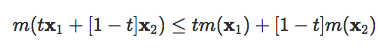Hidreth (1954) proved that if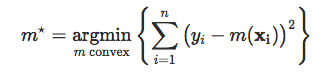then θ⋆=(m⋆(x1),⋯,m⋆(xn)) is unique.

Let y=θ+ε, then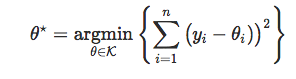where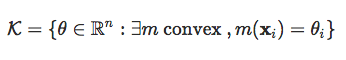I.e. θ is the projection of \mathbf{y}y onto the (closed) convex cone \mathcal{K}K. The projection theorem gives existence and unicity.

For convenience, in the application, we will consider the real-valued case, m:RR, i.e. yi=m(xi)+εi. Assume that observations are ordered x1≤x2≤⋯≤xn. Here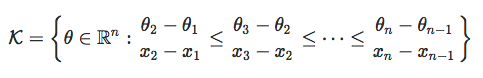Hence, quadratic program with n−2 linear constraints.

m is a piecewise linear function (interpolation of consecutive pairs (xi,θi⋆)).

If m is differentiable, m is convex ifMore generally, if m is convex, then there exists ξxRn such that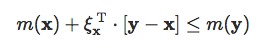ξx is a subgradient of m at x. And then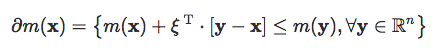Hence, θ is solution of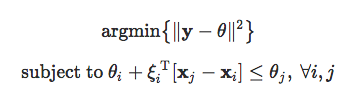and ξ1,⋯,ξnRn. Now, to do it for real, use cobs package for constrained (b)splines regression,

library(cobs)

To get a convex regression, use

plot(cars)
x = cars$speed y = cars$dist
rc = conreg(x,y,convex=TRUE)
lines(rc, col = 2)Here we can get the values of the knots

rc

Call:  conreg(x = x, y = y, convex = TRUE)
Convex regression: From 19 separated x-values, using 5 inner knots,
7,    8,    9,   20,   23.
RSS =  1356; R^2 = 0.8766;
needed (5,0) iterations

and actually, if we use them in a linear-spline regression, we get the same output here

reg = lm(dist~bs(speed,degree=1,knots=c(4,7,8,9,,20,23,25)),data=cars)
u = seq(4,25,by=.1)
v = predict(reg,newdata=data.frame(speed=u))
lines(u,v,col="green")

Let us add vertical lines for the knots

abline(v=c(4,7,8,9,20,23,25),col="grey",lty=2)Managing data at scale doesn’t have to be hard. Find out how the completely free, open source HPCC Systems platform makes it easier to update, easier to program, easier to integrate data, and easier to manage clusters. Download and get started today.

Topics:
big data ,convex regression model ,data modeling

Comment (0)

Save
{{ articles.views | formatCount}} Views

Opinions expressed by DZone contributors are their own.

# {{ parent.title || parent.header.title}}

{{ parent.tldr }}

{{ parent.urlSource.name }}